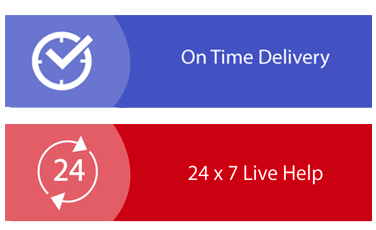× Limited Time Offer ! FLAT 30-40% off - Grab Deal Before It’s Gone. Order Now

## Statistical Analysis Assignment Sample

### INSTRUCTIONS

Perform the required calculations using Excel (and PHStat where appropriate), present your findings (i.e., the relevant output), and prepare short written responses to the following questions. Please note that you must provide a clear interpretation and explanation of the results reported in your output. Please submit your answers in a single Word file.

### Question 1 – Binomial Distribution (8 marks)

A university has found that 2.5% of its students withdraw without completing the introductory business analytics course. Assume that 100 students are registered for the course.

a) What is the probability that two or fewer students will withdraw?
b) What is the probability that exactly five students will withdraw?
c) What is the probability that more than three students will withdraw?
d) What is the expected number of withdrawals from this course?

### Question 2 – Normal Distribution

Suppose that the return for a particular investment is normally distributed with a population mean of 10.1% and a population standard deviation of 5.4%.
a) What is the probability that the investment has a return of at least 20%?
b) What is the probability that the investment has a return of 10% or less?
A person must score in the upper 5% of the population on an IQ test to qualify for a particular occupation.
c) If IQ scores are normally distributed with a mean of 100 and a standard deviation of 15, what score must a person have to qualify for this occupation

### Question 3 – Normal Distribution (4 marks)

According to a recent study, the average night’s sleep is 8 hours. Assume that the standard deviation is 1.1 hours and that the probability distribution is normal.
a) What is the probability that a randomly selected person sleeps for more than 8 hours?
b) Doctors suggest getting between 7 and 9 hours of sleep each night. What percentage of the population gets this much sleep?

### Question 4 – Normal Distribution (10 marks)

The time needed to complete a final examination in a particular college course is normally distributed with a mean of 160 minutes and a standard deviation of 25 minutes. Answer the following questions:

a) What is the probability of completing the exam in 120 minutes or less?
b) What is the probability that a student will complete the exam in more than 120 minutes but less than 150 minutes?
c) What is the probability that a student will complete the exam in more than 100 minutes but less than 170 minutes?
d) Assume that the class has 120 students and that the examination period is 180 minutes in length. How many students do you expect will not complete the examination in the allotted time?

## Solution

### Question 1

Here, number of trials (n) = 100
Probability of success i.e. student withdrawing from course (p) = 0.025
The various probabilities have been computed using BINOMIST function in Excel.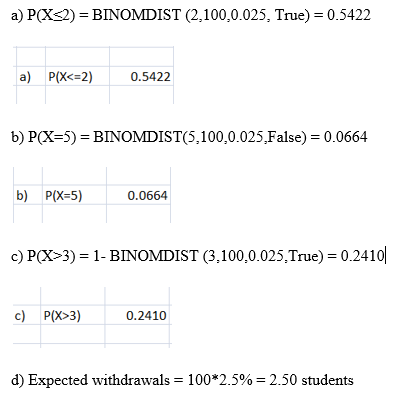### Question 2

a) Mean = 10.1%
Standard deviation = 5.4%
The relevant output from Excel is shown below.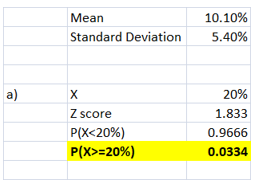The z score is computed using the inputs provided. Using the NORMS.DIST(1.833) function, the probability of P(X<20%) has been determined. Finally, the probability that the return would be atleast 20% is 0.0334.

b) Mean = 10.1%
Standard deviation = 5.4%
The relevant output from Excel is shown below for assignment help

The z score is computed using the inputs provided. Using the NORMS.DIST(-0.0185) function, the probability of P(X<10%) has been determined. It can be concluded that there is a probability of 0.4926 that the given investment has a return of 10% or less.

c) The relevant output from Excel is shown below.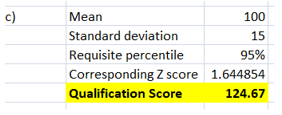The requisite percentile score required is 95%. The corresponding Z value for this as determined using NORMS.INV has come out as 1.644854. This along with the mean and standard deviation has been used to derive the minimum qualification score as 124.67. Hence, to be in the top 5%, a candidate needs to score atleast 124.67 in the IQ test.

### Question 3

a) The relevant output from Excel is shown below.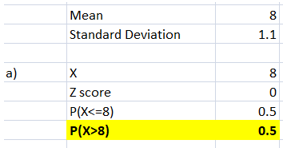Using the mean, standard deviation and the X value, the Z score has been computed as zero. Using NORMS.DIST(0) function, the probability of P(X<=8) has come out as 0.5. Further, the probability of P(X>8) has been computed as 0.5. Hence, the probability that a randomly selected person sleeps more than 8 hours is 0.5.

b) The relevant output from Excel is shown below.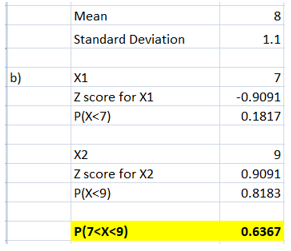For X1 = 7 hours, the corresponding z score was computed followed by finding the P(X<7) using NORMS.DIST(-0.9091) function. For X2 = 9 hours, the corresponding z score was computed followed by finding the P(X<9) using NORMS.DIST(0.9091) function. The probability that people would be getting sleep between 7 and 9 hours is 0.6367. Thus, 63.67% of the population is getting sleep between the 7 and 9 hours each night.

### Question 4

a) The relevant output from Excel is shown below.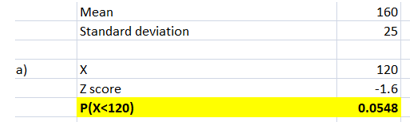For X =120, the corresponding z score was computed followed by finding the P(X<=120) using NORMS.DIST(-1.6) function. The probability of completing the exam in 120 minutes or lesser is 0.0548.

b) The relevant output from Excel is shown below.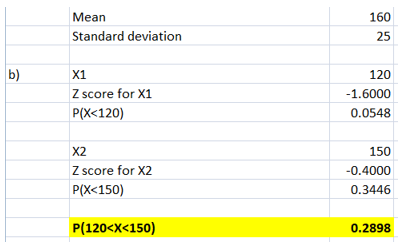For X1 = 120 minutes, the corresponding z score was computed followed by finding the P(X<120) using NORMS.DIST(-1.6) function. For X2 = 150 minutes, the corresponding z score was computed followed by finding the P(X< 150) using NORMS.DIST(-0.40) function. The probability that a student will complete the test in more than 120 minutes but less than 150 minutes is 0.2898.

c) The relevant output from Excel is shown below.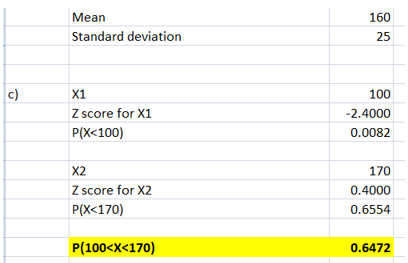For X1 = 100 minutes, the corresponding z score was computed followed by finding the P(X<100) using NORMS.DIST(-2.4) function. For X2 = 170 minutes, the corresponding z score was computed followed by finding the P(X< 170) using NORMS.DIST(0.40) function. The probability that a student will complete the test in more than 100 minutes but less than 170 minutes is 0.6472.

d) The relevant output from Excel is shown below.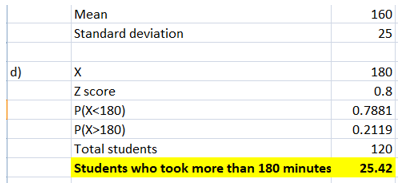For the score of 180 minutes, the corresponding Z score is 0.8. Using NORMS.DIST(0.8), the P(X<180) is 0.7881. Hence, the probability of student taking more than 180 minutes or not finishing within the allotted 180 minutes is 0.2119. The total number of students given is 120. Thus, students not finishing within the allotted 180 minutes is 0.2119*120 = 25.42 or 26 students.

Fill the form to continue reading